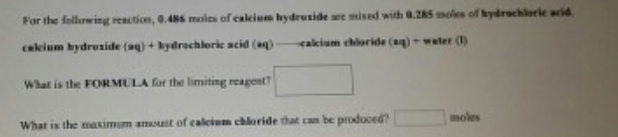# Problem: For the following reaction, 0.486 moles of calcium hydroxide are mixed with 0.285 moles of hydrochloric acid.calcium hydroxide(aq) + hydrochloric acid(aq) → calcium chloride(aq) + water(l) What is the FORMULA for the limiting reagent? What is the maximum amount of calcium chloride that can be produced?

###### FREE Expert Solution
91% (464 ratings)###### Problem Details

For the following reaction, 0.486 moles of calcium hydroxide are mixed with 0.285 moles of hydrochloric acid.

calcium hydroxide(aq) + hydrochloric acid(aq) → calcium chloride(aq) + water(l)

What is the FORMULA for the limiting reagent?

What is the maximum amount of calcium chloride that can be produced?